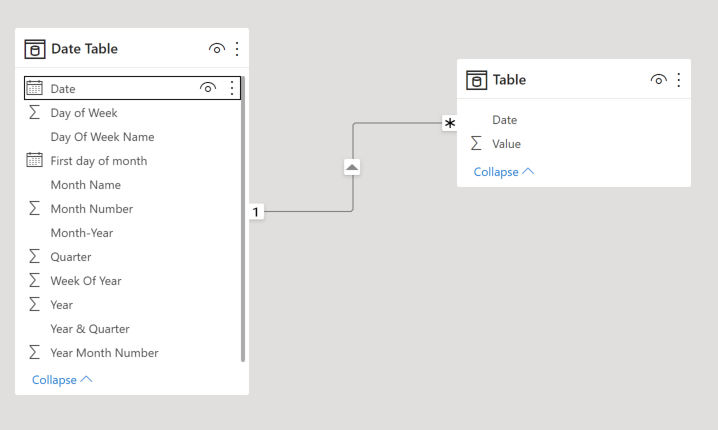top of page
Search
• Vahid

# WEEKLY TIME INTELLIGENCE CALCULATIONS IN DAXData Analysis Expressions (DAX) includes time-intelligence functions that enable you to manipulate data using time periods, including days, months, quarters, and years, and then build and compare calculations over those periods. Still, weeks are not part of those functions!

This article will show you how to create time intelligence functions using weeks period, like PREVIOUSWEEK, DATESWTD, ENDOFWEEK, STARTOFWEEK, Week Over Week, Rolling weeks, Same Week Last Year.

First of all, to work with Data Analysis Expressions (DAX) time intelligence functions, there's a prerequisite model requirement: You must have at least one Date table in your model. Click on the following link to create a Date Table with three steps : Creating Calendar Table with Power Query M Function

After adding the date table, I added another table with two columns {"Date", "Value"} to the data model and created a relationship between date columns:Now or model is ready to create weekly time intelligence functions, I added a question and two answers for each weekly function, one with the Date Table and one without the Date Table, to make it more understandable.

### PREVIOUSWEEK

##### Question: What is the total value of the previous week?

With Date Table: PREVIOUSWEEK Value = VAR _Today = TODAY() VAR _WOT = WEEKNUM( _Today, 2 ) -- Number 2 determines that the week begins on Monday. VAR _LW = _WOT - 1 RETURN CALCULATE( SUM( 'Table'[Value] ), FILTER( ALL( 'Date Table' ), 'Date Table'[Week Of Year] = _LW && 'Date Table'[Year] = YEAR( TODAY() ) ) ) Without Date Table: PREVIOUSWEEK Value WDT = VAR _Today = TODAY() VAR _WOT = WEEKNUM( _Today, 2 ) -- Number 2 determines that the week begins on Monday. VAR _LW = _WOT - 1 RETURN CALCULATE( SUM( 'Table'[Value] ), FILTER( ALL( 'Table' ), WEEKNUM( 'Table'[Date], 2 ) = _LW && YEAR( 'Table'[Date] ) = YEAR( TODAY() ) ) )

### DATESWTD

##### Question: What is the Week To Date total value?

With Date Table: DATESWTD Value = VAR _Today = TODAY() VAR _WOT = WEEKNUM( _Today, 2 ) -- Number 2 determines that the week begins on Monday. RETURN CALCULATE( SUM( 'Table'[Value] ), FILTER( ALL( 'Date Table' ), 'Date Table'[Week Of Year] = _WOT && 'Date Table'[Year] = YEAR( TODAY() ) ) )

Without Date Table: DATESWTD Value WDT = VAR _Today = TODAY() VAR _WOT = WEEKNUM( _Today, 2 ) -- Number 2 determines that the week begins on Monday. RETURN CALCULATE( SUM( 'Table'[Value] ), FILTER( ALL( 'Table' ), WEEKNUM( 'Table'[Date], 2 ) = _WOT && YEAR( 'Table'[Date] ) = YEAR( TODAY() ) ) )

### ENDOFWEEK

##### Question: How to add a column to my table to show the last date of the week for each date column value (or any other column with date value/format)?

ENDOFWEEK = VAR _WD = WEEKDAY( 'Table'[Date], 2 ) -- Number 2 determines that the week begins on Monday; change it to 1 if you want week starts on Sunday (1) and ends on Saturday (7) RETURN 'Table'[Date] + ( 7 - _WD )### STARTOFWEEK

##### Question: How to add a column to my table to show the last date of the week for each date column value (or any other column with date value/format)?

STARTOFWEEK = VAR _WD = WEEKDAY( 'Table'[Date], 2 ) -- Number 2 determines that the week begins on Monday; change it to 1 if you want week starts on Sunday (1) and ends on Saturday (7) RETURN 'Table'[Date] - _WD +1### Week Over Week (WOW%)

##### Question: How to show % of week over week increase in Value?

WOW% = VAR _Date = MAX( 'Table'[Date] ) VAR _WOT = WEEKNUM( _Date, 2 ) -- Number 2 determines that the week begins on Monday. VAR _LW = _WOT - 1 VAR _LWV = CALCULATE( SUM( 'Table'[Value] ), FILTER( ALL( 'Date Table' ), 'Date Table'[Week Of Year] = _LW && 'Date Table'[Year] = YEAR( TODAY() ) ) ) VAR _TWV = SUM( 'Table'[Value] ) RETURN ( _TWV - _LWV ) / _LWV

### Rolling weeks

##### Question: How to calculate the total value for the previous 12 weeks?

Rolling 12 Weeks = VAR _Date = MAX ( 'Table'[Date] ) VAR _WOT = WEEKNUM ( _Date, 2 ) -- Number 2 determines that the week begins on Monday. VAR _StartofEWeek = CALCULATE ( MIN ( 'Date Table'[Date] ), FILTER ( ALL ( 'Date Table' ), 'Date Table'[Week Of Year] = _WOT && 'Date Table'[Year] = YEAR ( _Date ) ) ) -- the first day of the end week VAR _NumberofWeek = 12 -- Number of the rolling weeks, we want to calculate the Rolling 12 Weeks so this is 12 now VAR _DaysBetween = ( _NumberofWeek - 1 ) * 7 VAR _StartofSweek = _StartofEWeek - _DaysBetween -- first day of the start week RETURN CALCULATE ( SUM ( 'Table'[Value] ), FILTER ( ALL ( 'Date Table' ), 'Date Table'[Date] >= _StartofSweek && 'Date Table'[Date] <= _Date ) )

### Same Week Last Year

##### Question: What was the total value of the same week last year?

Same Week Last Year = VAR _Date = MAX ( 'Table'[Date] ) VAR _WOT = WEEKNUM ( _Date, 2 ) -- Number 2 determines that the week begins on Monday. VAR _LY = YEAR ( _Date ) - 1 RETURN CALCULATE ( SUM ( 'Table'[Value] ), FILTER ( ALL ( 'Date Table' ), 'Date Table'[Week Of Year] = _WOT && 'Date Table'[Year] = _LY ) )

Without Date Table: Same Week Last Year WDT = VAR _Date = MAX ( 'Table'[Date] ) VAR _WOT = WEEKNUM ( _Date, 2 ) -- Number 2 determines that the week begins on Monday. VAR _LY = YEAR ( _Date ) - 1 RETURN CALCULATE ( SUM ( 'Table'[Value] ), FILTER ( ALL( 'Table' ), WEEKNUM( 'Table'[Date], 2 ) = _WOT && YEAR( 'Table'[Date] ) = _LY ) ) Download the Sample File:

Sample File
.rar